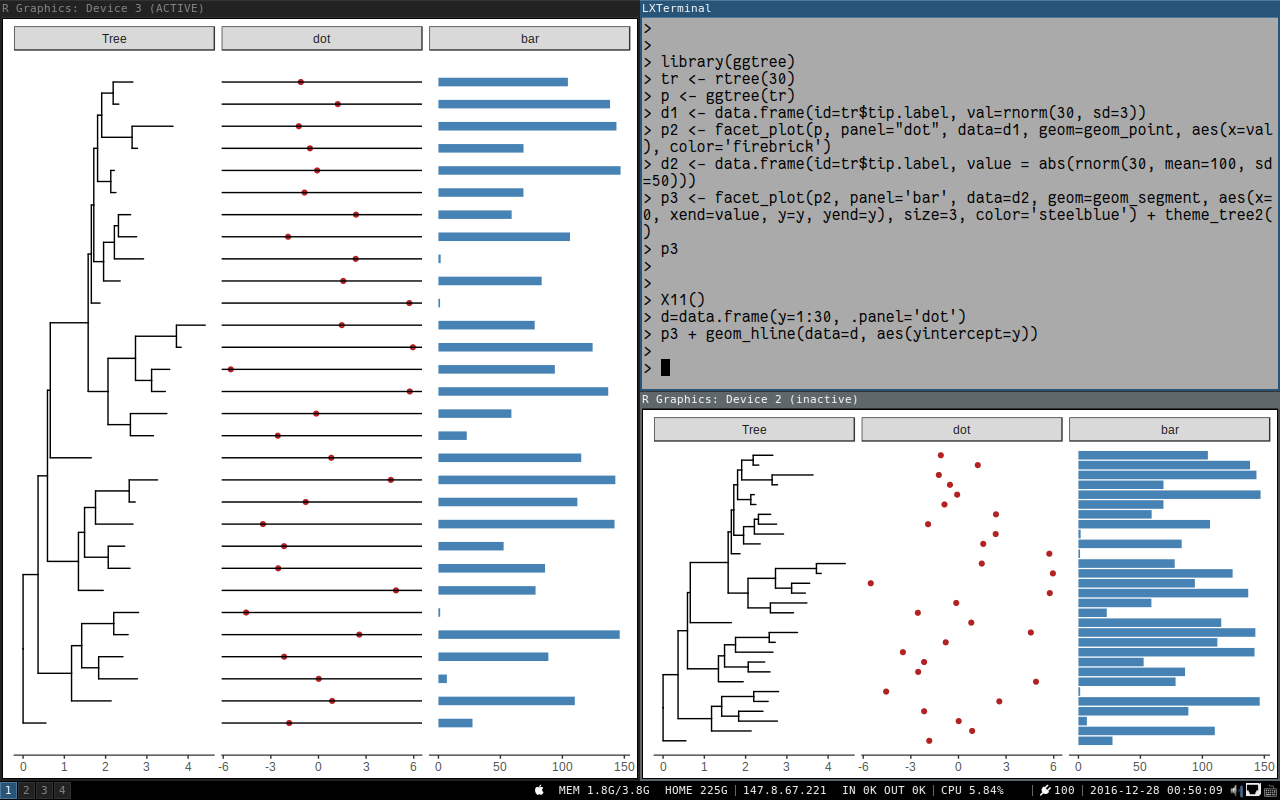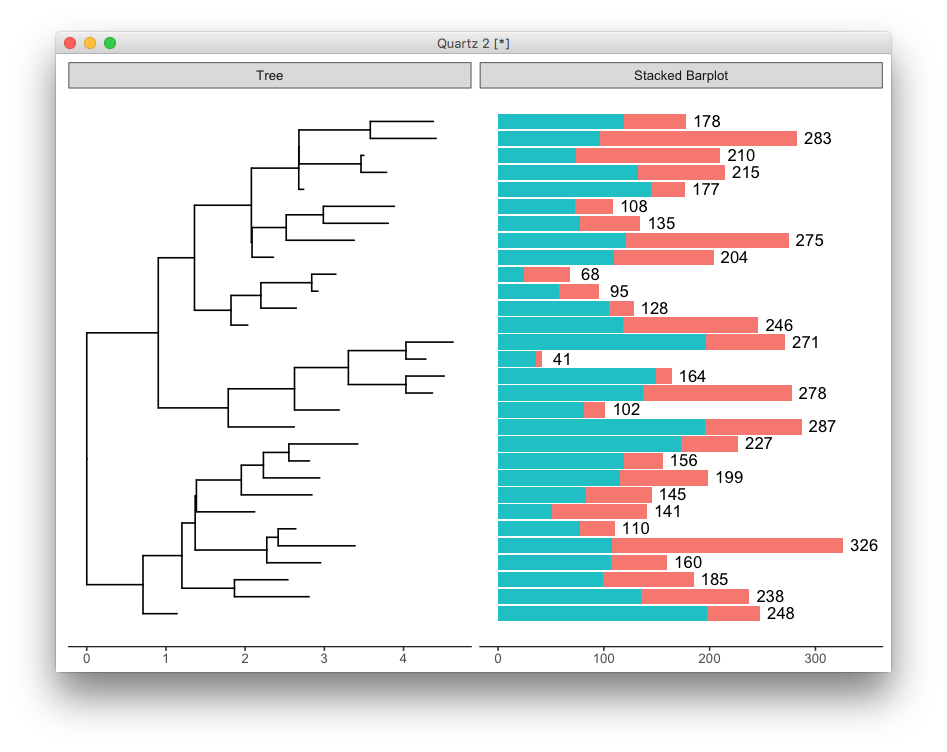This is a question from ggtree google group:

Dear ggtree team,

how can I apply a geom_xxx to only one facet panel? For example if i want to get `geom_hline(yintersect=1:30)` or a `geom_text()` in the dot panel? I cant see the `facet_grid(. ~ var)` function call, so I don’t know which subsetting to use. I have already read http://stackoverflow.com/questions/29873155/geom-text-and-facets-not-working

``````  tr <- rtree(30)

d1 <- data.frame(id=tr\$tip.label, val=rnorm(30, sd=3))
p <- ggtree(tr)

p2 <- facet_plot(p, panel="dot", data=d1, geom=geom_point, aes(x=val), color='firebrick')
d2 <- data.frame(id=tr\$tip.label, value = abs(rnorm(30, mean=100, sd=50)))

p3 <- facet_plot(p2, panel='bar', data=d2, geom=geom_segment, aes(x=0, xend=value, y=y, yend=y), size=3, color='steelblue') + theme_tree2()
``````

Thanks! Andreas

If this can be done, we can create even more comprehensive tree plots.

In fact, it’s quite easy to do. In `facet_plot`, `ggtree` used a variable `.panel` to store the panel information. We can add a dummy column `.panel` to our data and pass it to `geom` layer function and the layer will be added to selected panel we specify in the `.panel` column.

To follow up the above example, we can create a `data.frame` that contains `y` and `.panel="dot"` as we want to add horizontal lines in `dot` panel.

``````d=data.frame(y=1:30, .panel='dot')
p3 + geom_hline(data=d, aes(yintercept=y))
``````

Here is what we can get:The xlim_expand is actually adding a `geom_blank` layer to selected panel for setting x-axis limits of that panel.

Another approach is using `facet_plot` with panel parameter setting to existing one.

An example is posted in google group:

``````library(dplyr)
library(ggstance)
library(ggtree)

tr <- rtree(30)
p <- ggtree(tr) + theme_tree2()

df <- data.frame(id = rep(tr\$tip.label, each=2),
value = abs(rnorm(60, mean=100, sd=50)),
category = rep(LETTERS[1:2], 30))

sum_value <- df %>%
group_by(id) %>%
summarize(total = sum(value))

p2 <- facet_plot(p, panel = 'Stacked Barplot',
data = df, geom = geom_barh,
mapping = aes(x = value, fill = as.factor(category)),
stat='identity' )

facet_plot(p2, panel='Stacked Barplot',
data=sum_value, geom=geom_text,
mapping=aes(x=total+20, label=round(total)))
``````## Citation

G Yu, DK Smith, H Zhu, Y Guan, TTY Lam*. ggtree: an R package for visualization and annotation of phylogenetic trees with their covariates and other associated data. Methods in Ecology and Evolution. `doi:10.1111/2041-210X.12628`.